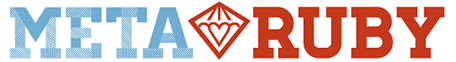# Delete every other item in the array until only one is left

Hello everybody,

My problem is asking to iterate over an array of items and delete every other item until I reach the end of the array when I should start iterating backwards and keep deleting every other item and so on back and forth until only one item is left in the array.

for example: 1,2,3,4,5, would become 1,3,5 and then 3

I understand that I shouldn’t be deleting from the original array so I created another array that just keeps every other item while decreasing its size but I can’t get it to work and end up with an infinite loop.

``````arr=*(1..10)
s_arr=[]
until s_arr.length==1
i=0
while i<arr.length
s_arr.push(arr[i])
i+=2
end
arr=s_arr.reverse
s_arr=arr
end
``````

Any help would be greatly appreciated.
God Bless

You could reject every other element from the array with `reject` and `with_index`.

``````arr = *(1..10)
until arr.size <= 1
arr = arr.reject.with_index { |_, idx| idx.odd? }
end
``````

This will iterate through the array, the `_` here means a variable I don’t care about (convention when you do stuff like this, it is actually the current element) since we only care about the index being either even or odd. We start from `0` index, so we reject every other by rejecting the odd numbers. We overwrite the original array, and then look until the array is size `1`.

@Ohm Thank you very much for your reply. I don’t understand it completely. Does your code iterate through the array both way? The requirement of the problem is to iterate left to right and right to left until the end when I’m left with only one element.

Ahh… Sorry, didn’t read that part.I was wondering what the `reverse` was doing in there.

No, the above solution of course only rejects every other element from left to right.

Extending my program from above, you could reverse the array afterwards, so having

``````arr = *(1..10)
until arr.size <= 1
arr = arr.reject.with_index { |_, idx| idx.odd? }.reverse
end
``````

This would reject all the odd indices and then flip the array until only one element remains.

1 Like

Brilliant! Thanks!
I got mine to work finally but it looks like Frankenstein when I compare it to your code.

``````def arr_redux(arr)
s_arr=[]

until s_arr.length == 1
i=0
while i < arr.length
s_arr<<arr[i]
i+=2
end
arr=s_arr.reverse
if s_arr.length != 1
s_arr=[]
end
end
end
``````

@Ohm I’m unable to grasp completely the underscore meaning. I know you said it refers to a variable that we don’t really care about. Could you elaborate please? Or maybe provide another simple example when using the underscore would be useful? Thanks

The use of underscore as a placeholder is just the convention. It doesn’t have any significance in itself. We could just as easily have used `var`, `i` or some other variable name.

This:

``````arr = *(1..10)
until arr.size <= 1
arr = arr.reject.with_index { |elem, idx| idx.odd? }.reverse
end
``````

would work just the same. The reason I choose `_` is just to say “I know that we get the element as well, but since I’m not using it, I will not name it.”

Does that make sense?

Ok so we are not using the element in our evaluation but it does get rejected along with the index right? In other words it is mandatory that we have 2 arguments inside the block because we need to remove both of them simultaneously otherwise the code wouldn’t work. Am I understanding this correctly?

Yes, the `with_index` is a bit magic here.

Let me try to show by example what is actually going on:

First lets look at `map`. `map` iterates through the array and returns the result in the same place. So if we do `elem + 1` we should end up with an array where each element have been increased by one.

``````arr = [1, 2, 3, 4, 5, 6, 7, 8, 9, 10]
arr.map { |elem| elem + 1 } # => [2, 3, 4, 5, 6, 7, 8, 9, 10, 11]
``````

We can now look at `select`, which as the name suggests selects the elements and keep those we need. That is, if the block evaluate to `true` we keep the element else we discard it.

``````arr = [1, 2, 3, 4, 5, 6, 7, 8, 9, 10]
arr.select { |elem| elem == 5 } # => 
arr.select { |elem| elem > 7 } # => [8, 9, 10]
``````

`reject` is just the opposite of `select`. Here we reject, if we evaluate to `true`

``````arr = [1, 2, 3, 4, 5, 6, 7, 8, 9, 10]
arr.reject { |elem| elem == 5 } # => [1, 2, 3, 4, 6, 7, 8, 9, 10]
arr.reject { |elem| elem > 7 } # => [1, 2, 3, 4, 5, 6, 7]
``````

It is important to note that all of these methods return a new array and thus do not change the original.

`with_index` works on an enumerator, which `map`, `select` and `reject` (among others) return. So if we call `with_index` on the `reject` directly, without giving a block to `reject` we get the magic `with_index` construct. This will call the original enumerator (e.g. `select` or `reject`) but will yield both the element and the current index.

You can thus think of the `with_index` as changing your array into an array of pairs:

``````arr =[(1,0), (2,1), (3,2), (4,3), (5,4), (6,5), (7,6), (8,7), (9,8), (10,9)]
``````

and if we call `reject` on these, we get a pair out, thus the two arguments. Since we only care about the index, and not the actual value of the element, we can ignore the first argument, which as a codestyle you show with `_`.

1 Like

You are a Gentleman and a Scholar! Great explanation. Thank you!

1 Like